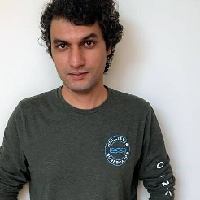TensorFlow (Intermediate): Linear Regression

In this course, we are going to solve a linear regression problem with the help of TensorFlow and in the eager execution mode of TensorFlow. Let’s say we have an equation like this: `y = Wx + b`. And let’s say we have a lot of values for `y` and `x`. Given that, can we find out the values for `W` and `b`? This is a basic machine learning problem and this is pretty much the fundamental concept of how we make machines learn. Essentially, we just make machines find the optimal values for parameters (like `W` and `b` in this case), given an assumed model and a bunch of data. So, we try to find the values for parameters which will best fit the data that we have.Duration (mins)

Learners

5.0 / 5

Rating

We will cover the following tasks in 36 minutes:

Introduction

Normally, when you use TensorFlow to create and train machine learning models, you need to build computational graphs first required for your model training first and then run those graphs later to actually perform the computations. However, this approach is not very easy or intuitive to use. In production setting, this may not be a big problem but if you’re just researching and experimenting with your potential models, then this traditional approach can slow things down.

This is where eager execution comes in. TensorFlow’s eager execution facilitates an imperative programming environment that allows the programmer to evaluate operations immediately, instead of first creating computational graphs to run later.

Data Synthesis

We are going to synthesise our data which we will use to solve a linear regression problem. Now, usually you will get this data from observation but we are just learning here. So, for our purpose we will generate this data.

A good model will have values for `W` and `b` such that the cost on the entire set is minimized. We can say that a good indication of one single prediction being a good prediction would be that the squared difference between the prediction and the actual value for `y` for the same `x` is low. If this value is high, then the prediction is obviously not good.

And an indication of a good model would be if these loss values for all values for `x`, put together, is low. This is called cost of a model. But how do we know if a cost is low or high?

We can use an algorithm called gradient descent which starts off with some arbitrary values for `W` and `b` and then we calculate the gradients of our cost function with respect to these values. We then take a small step in the direction of these slopes to, hopefully, move closer to a minima.

Linear Regression Model

Based on our understanding so far, we will need to define four functions in our Linear Regression Model: the initializer where we set the initial values for model’s parameters, a cost function, a predict function and a train function.

The predict function will take a inputs and predict outputs based on latest values of parameters `W` and `b`. The cost function will take this prediction and the actual outputs and will calculate the cost J based on that.

The Training Loop

The train function will take an inputs and outputs and will run a loop for a few iterations that we can decide beforehand. In each iteration, the values for `W` and `b` will be updated.

Training the Model

We have actually done bulk of the work in the previous chapter and now we just need to call the train method (defined previously) after instantiating our model. This will fit out model to the given data, giving us a model with optimal values for our parameters!

Watch Preview

Preview the instructions that you will follow along in a hands-on session in your browser.

Reviews

There should be a way to get some quick questions answered..Sharma KunapalliI am a Software Engineer with many years of experience in writing commercial software. My current areas of interest include computer vision and sequence modelling for automated signal processing using deep learning as well as developing chatbots.

How is this different from YouTube, PluralSight, Udemy, etc.?
In Rhyme, all projects are completely hands-on. You don't just passively watch someone else. You use the software directly while following the host's (Amit) instructions. Using the software is the only way to achieve mastery. With the "Live Guide" option, you can ask for help and get immediate response.